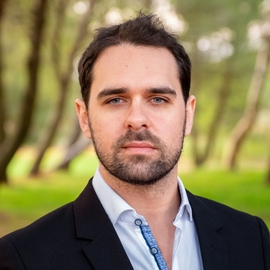# Gaussian process optimization for self-tuning control

### Abstract

Robotic setups often need fine-tuned controller parameters both at low- and task-levels. Finding an appropriate set of parameters through simplistic protocols, such as manual tuning or grid search, can be highly time-consuming. This thesis proposes an automatic controller tuning framework based on linear optimal control combined with Bayesian optimization. With this framework, an initial set of controller gains is automatically improved according to the performance observed in experiments on the physical plant. In the tuning scenario that we propose, we assume we can measure the performance of the control system in experiments through an appropriate cost. However, we only allow a limited number of experimental evaluations (e.g. due to their intrinsic monetary cost or effort). The goal is to globally explore a given range of controller parameters in an efficient way, and return the best known controller at the end of this exploration. At each iteration, a new controller is generated and tested on a closed-loop experiment in the real plant. Then, the recorded data is used to evaluate the system performance using a quadratic cost. We re-iterate in order to solve a global optimization problem, whose goal is to learn most about the location of the global minimum from the limited number of experiments. We use the Linear Quadratic Regulator (LQR) formulation as a standard way to compute optimalmultivariate controllers given a linear plant model and quadratic cost. We parametrize the LQR weights in order to obtain controllers with appropriate robustness guarantees. The underlying Bayesian optimization algorithm is Entropy Search (ES), which represents the latent objective as a Gaussian process and constructs an explicit belief over the location of the objective minimum. This method maximizes the information gain from each experimental evaluation. Thus, this framework shall yield improved controllers with fewer evaluations compared to alternative approaches. A seven-degree-of-freedomrobot arm balancing an inverted pole is used as the experimental demonstrator. Results of a two-dimensional tuning problem are shown in two different contexts: in the first setting, a wrong linear model is used to compute a nominal controller, which destabilizes the actual plant. The automatic tuning framework is still able to find a stabilizing controller after a few iterations. In the second setting, a fairly good linearmodel is used to compute a nominal controller. Even then, the framework can still improve the initial performance by about 30%. In addition, successful results on a four-dimensional tuning problem indicate that the method can scale to higher dimensions. Themain and novel contribution of this thesis is the development of an automatic controller tuning framework combining ES with LQR tuning. Albeit ES has been tested on simulated numerical optimization problems before, this work is the first to employ the algorithm for controller tuning and apply it on a physical robot platform. Bayesian optimization has recently gained a lot of interest in the research community as a principled way for global optimization of noisy, black-box functions using probabilistic methods. Thus, this work is an important contribution towardmaking thesemethods available for automatic controller tuning for robots. In conclusion, we demonstrate in experiments on a complex robotic platform that Bayesian optimization is useful for automatic controller tuning. Applying Gaussian process optimization for controller tuning is an emerging novel area. The promising results of this work open up many interesting research directions for the future. In future work, we aim at scaling this framework further than this problem to higher dimensional systems such as balancing of a full humanoid robot for which a rough linear model can be obtained. In addition, comparing ES with other global optimizers from the literature may be of interest. Investigating safety considerations such as avoiding unstable controllers during the search is also part of future research.